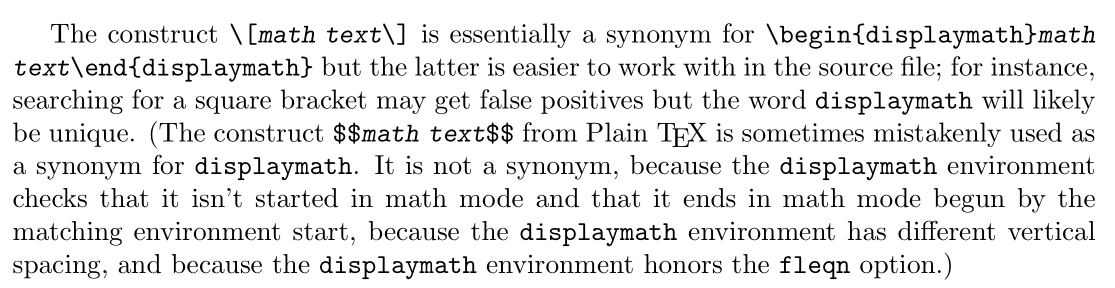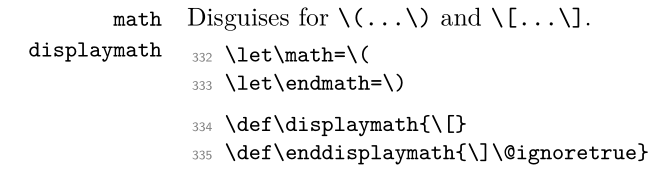# LaTeX Convention for displaymath

Welcome to TEX.SE! You can first see this answer.

If you search "displaymath" in texdoc latex2e and you can find in sec 8.5:Further more if you search "displaymath" in texdoc source2e, you can find thatYou can see in LaTeX, $mathtext$ is equal to \begin{displaymath} mathtext \end{displaymath}. If you write your source file like this

$mathtext$
\begin{displaymath}
mathtext
\end{displaymath}


both of them seems clean and can remaind you these are "displaymath".

When you use amsmath package, it redefines $mathtext$ as \begin{equation*} mathtext \end{equation*} for numberless equation. (You can see this in amsmath.sty)